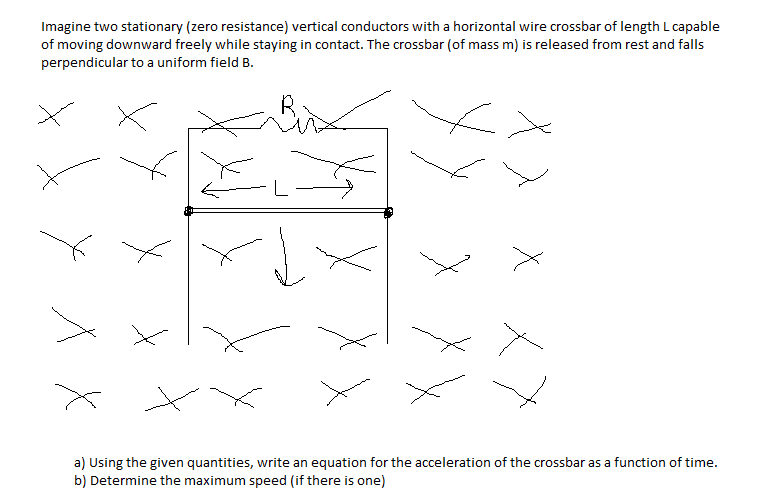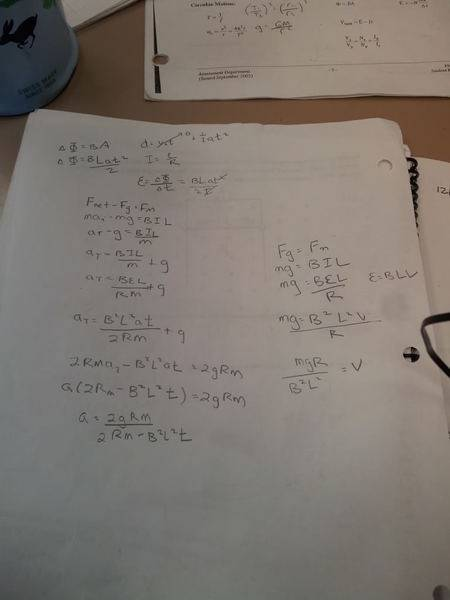# Electromagnetism question involving two stationary conductors and crossbars

## Homework Statement

Question and diagram below## Homework Equations

F=BIL
F=QVB
Vback=(epsilon)-Ir

## The Attempt at a Solution

F=QvB
ma=QvB
QvB/m=a ?
I really don't know how to start this question.

## Answers and Replies

Hi mrcheeses. The question says that "the bar is released and fall", so is the bar under the influence of gravity or not? Also for this situation you should use $F_B=BIL$ for the force on the bar due to the magnetic field. You can find the current flowing through the bar using Faraday's law.

I don't think gravity effects it, but I found out that the diagram is of a rail gun.

Nevermind about gravity. Im not really sure but I think you have to account for it.

I just tried doing this again and my work is below...

V=IR
I=V/R
F=BIL
Fnet=Fg-F
ma=mg-BIL
ma=mg-BVL/r
a=(mg-BVL/r)/m

Not sure if this is right but its not as a function of time!

ehild
Homework Helper
How do you get V, the emf in the circuit? What happens if the magnetic flux enclosed by a loop changes in time? Does it change when the crossbar falls?

ehild

I just got V from the circuits formula V=IR but I guess you can only do that in a DC current.

If the magnetic flux changes in time, that means the area would change or the magnetic field would change.

The magnetic flux would change when the crossbar falls because there will be a greater area.

So to get the equation, would I have to start off with F=BIl and since Fnet=ma, ma=BIl and then incorporate time in it somehow, or would I have to start with ε=-NΔΦ/Δt?

ehild
Homework Helper
So to get the equation, would I have to start off with F=BIl and since Fnet=ma, ma=BIl and then incorporate time in it somehow, or would I have to start with ε=-NΔΦ/Δt?

You need both equations. Faraday's law of induction gives you the unknown emf in the circuit from what you find the current as I=ε/R. From the current, you get the force of the magnetic field on the crossbar. From Lenz's law you know that the induced emf works against the effect that produced it. The resultant of the magnetic force and gravity determines the acceleration of the bar.

ehild

So what I would do is use F=BIL and then solve for I and substitute it in for I in I=ε/R.

Then get ε on one side which gives

ε=FR/BL

and then substitute Faradays-Lenz law for ε which gives

-NΔΦ/Δt=FR/BL

Then make another equation for just forces.

Fnet= F+Fg
ma=F+mg
F=ma-mg

Sub it in for F and then do the math and get acceration on one side by itself which gives

mghΔt-NΔΦBL/ΔtmR=a

Is this right?

ehild
Homework Helper
mghΔt-NΔΦBL/ΔtmR=a

Is this right?

It is almost right, but you have not finished yet.

What is h? Why is it there in your formula?

What is N? Is it needed in this case?

The magnetic flux is related to B. How?
The time derivative of the magnetic flux is related to the velocity of the bar. How?

ehild

Sorry the h was a typo, it was supposed to be R. I guess you can just remove the N since there aren't coils wrapped around and we can consider it as one coil. Which gives

(mgRΔt-ΔΦBL)/ΔtmR=a

Magnetic Flux is related to magnetic field by Flux=FieldxArea, and since the area of a rail gun would change as the bar moves down, ΔΦ=BΔA so that gives

(mgRΔt-(B^2)AL)/ΔtmR=a

Would this be right?

And I don't get the last question, but I do not think I need calculus for my physics course so maybe my teacher just wanted us to include time in our equation.

Last edited:
ehild
Homework Helper
(mgRΔt-ΔΦBL)/ΔtmR=a

Magnetic Flux is related to magnetic field by Flux=FieldxArea, and since the area of a rail gun would change as the bar moves down, ΔΦ=BΔA so that gives

(mgRΔt-(B^2)ΔAL)/ΔtmR=a

Would this be right?
You left out Δ in front of A. So a=(mgRΔt-(B^2)ΔAL)/ΔtmR wich equals
a=(mgR-(B^2)L (ΔA/Δt))/mR

The area of the loop is A*y . y is the vertical length. As the bar falls, y increases. ΔA = LΔy. The velocity of the bar is v=Δy/Δt, so ΔA/Δt=Lv. Substitute for ΔA/Δt into the formula for the acceleration.
As the bar falls, its speed increases, but the magnetic force also increases, so the acceleration decreases with time. Can you find the velocity when the acceleration is zero?

ehild

Thanks for all the help!

I got mgR/B^2L^2=v when I did all the math. So would that be the answer for the second question?

ehild
Homework Helper
If you meant v=mgR/(B^2L^2) then it looks correct. Use parentheses.

ehild

Wait, for (mgRΔt-(B^2)ΔAL)/ΔtmR=a , would that work? If the change in time is 0, then the acceleration is undefined.

Would this work?Can you apply the kinematics equation into this since it is for constant acceleration?
The question says that the object falls from rest and with this solution, at t=0, a would =g and that would work since at t=0, the magnetic force would be 0 by F=qvb.

Last edited:
ehild
Homework Helper
Wait, for (mgRΔt-(B^2)ΔAL)/ΔtmR=a , would that work? If the change in time is 0, then the acceleration is undefined.

Can you apply the kinematics equation into this since it is for constant acceleration?
The question says that the object falls from rest and with this solution, at t=0, a would =g and that would work since at t=0, the magnetic force would be 0 by F=qvb.

I do not understand why the acceleration should be undefined at t=0.

I explained that ΔA=LvΔt. I do not know why you replaced ΔA by Lat. It is wrong. When you take into account that ΔA=LvΔt in the formula for acceleration you can simplify with Δt. Δt means a short time interval, it is not zero! There is no "t" in the formula.
You get (mgR-(B^2)vL^2)/mR=a. It is not constant acceleration!

At t=0, the acceleration is g as v=0. As the rod falls, its velocity increases, but the acceleration decreases. The velocity increases with smaller and smaller rate. The acceleration tends to zero with time and the velocity tends to the value v(max)=mgR/(B^2L^2) as you have figured out.

Using that a=dv/dt, the formula in blue means a differential equation for v. I quess you have not studied differential equations yet. The solution is of the form

v=v(max)(1-e-kt) with v(max)=mgR/(B2L2) and k=B2L2/(mR).

You can not apply the constant-acceleration formula v=at!

ehild

Okay great thanks for all the help! And Im sorry I copied and pasted the wrong formula. I meant to copy and paste a=(mgR-(B^2)L (ΔA/Δt))/mR.

ehild
Homework Helper
I am glad to be of help.

ehild